# Abstract Algebraic Examples and Going from a Graph to a Rule

An error occurred trying to load this video.

Try refreshing the page, or contact customer support.

Coming up next: Graphing Undefined Slope, Zero Slope and More

### You're on a roll. Keep up the good work!

Replay
Your next lesson will play in 10 seconds
• 0:04 What is an Abstract Equation?
• 0:38 Finding the Equation…
• 3:21 Identifying Graphs…
• 5:40 Finding the Greatest Slope
• 7:19 Solving Using Standard Form
• 9:57 Lesson Summary
Save Save

Want to watch this again later?

Timeline
Autoplay
Autoplay
Speed Speed Audio mode

#### Recommended Lessons and Courses for You

Lesson Transcript
Instructor: Ori Gold
Just because you now know what a linear equation is doesn't mean that you are a master! This video will help you learn how to apply your knowledge of linear equations in more abstract algebraic ways.

## What is an Abstract Equation?

In this lesson, we will do some problems that have to do with linear equations that are a little more abstract. Now, when I say abstract, I mean that they probably won't have very many numbers in them and there usually won't be a specific procedure you can follow to end up with the result they're looking for. These problems are usually a little bit harder and they're also a little bit harder to teach, but I'm hoping that these four examples we go through will give you an idea of what I'm talking about.

## Finding the Equation of a Graph

We'll start with a question that shows you a graph and asks you to write the rule for that graph. Looking at the graph, we can tell that it's a linear equation because it's a straight line and we know that any linear equation can be written as y = mx+b, where the m is the slope and the b is the y-intercept. So it's essentially our job to figure out those two things from the graph; what is the slope and what is the y-intercept?

It will often times be easiest to find the y-intercept first, but there's no particular order you need to do these things in. We'll go ahead and do the y-intercept first. We know that the y-intercept is the point where the graph crosses the y-axis. It's also the beginning point. In this case, the graph crosses the y-axis right here, which have the coordinates (0,3) because it's where x is zero and y is 3. This means that we already know the y-intercept for this graph, and it's 3. So we already have half the equation done. The only other thing left to find is the slope.Now there's a lot of different words and phrases for the slope; often 'rise over run' is used or 'change in y over change in x', often times represented with the little Delta symbols. But all that's meaning is that the slope is how much the line goes up and down divided by how much the line goes left and right. So we can pick two points - any two points on this graph - and simply connect them with a straight up and down line and a straight left and right line and count to see how much it's going up and down and left and right.

So maybe we'll pick this point and the y-intercept. I draw a straight up and down line and a straight left and right line to get there and we simply count. I started up here at 5 and I went down 2, and then I started at -3 and went over 3 to the right. This means that my slope, or my rise over run, or my change in y and change in x - just the amount I went up and down, which was -2 over the amount I went left and right - which was positive 3. I find that my slope is -2/3. This means the equation of my line is y = -2/3x+3.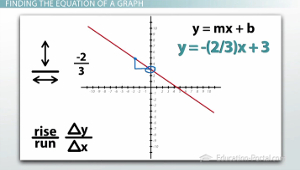## Identifying Graphs Based on Appearance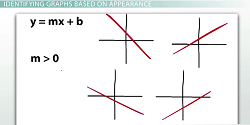This next question is a really good example of a more abstract question. It simply tells us that if we have a line that's written in y = mx+b form, and my m value is greater than zero (therefore it's positive) and my b value is less than zero (which makes it negative), which one of these graphs must my line be?

So we're not going to plug in any numbers and simply do a bunch of operations and eventually end up with an answer (what I might call 'plug and chug'). This question simply requires you to have an understanding of what m and b are at a conceptual level for you to answer it.

So we need to know that m is the slope, and the slope is the rise over the run again, and all those different things we mentioned, which means that as it goes to the right, it also goes up. So positive slopes go up from left to right; if you're trying to walk up it from left to right, you'd have to walk up the line. So we know that because my slope is positive, it has to go up from left to right, so we can rule out these two examples because they are both going down. These two examples would have slopes that are negative, so I know it must be one of the other two.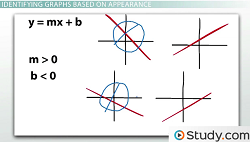Which brings us to our second clue that b is negative. So we have to remember that b is our y-intercept. It's the beginning value. It's the point where the line crosses the y-axis. And if b is negative, that means it crosses the y-axis below the x-axis. It crosses it down at the bottom of the graph somewhere. This means that because this graph has a y-intercept above the x-axis, in the positive part of the y-axis, this can't be it, which brings us to our answer right here. It has a positive slope, it's going up from left to right and it begins on the negative part of the y-axis.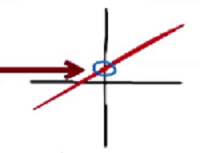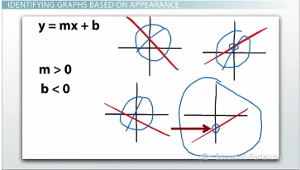## Finding the Greatest Slope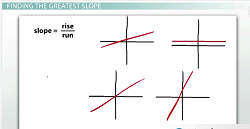Our third example, again has no numbers, and simply asks us which of the four graphs shown has the greatest slope. Again, we simply need to know a conceptual understanding of what slope is - rise over run, change in y over change in x, how much it goes up and down over how much it goes left and right.

To unlock this lesson you must be a Study.com Member.

### Register to view this lesson

Are you a student or a teacher?

#### See for yourself why 30 million people use Study.com

##### Become a Study.com member and start learning now.
Back
What teachers are saying about Study.com

### Earning College Credit

Did you know… We have over 200 college courses that prepare you to earn credit by exam that is accepted by over 1,500 colleges and universities. You can test out of the first two years of college and save thousands off your degree. Anyone can earn credit-by-exam regardless of age or education level.